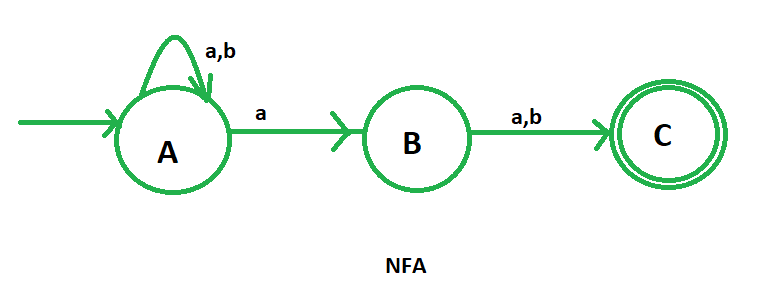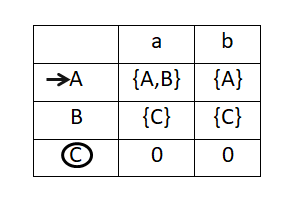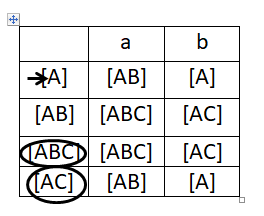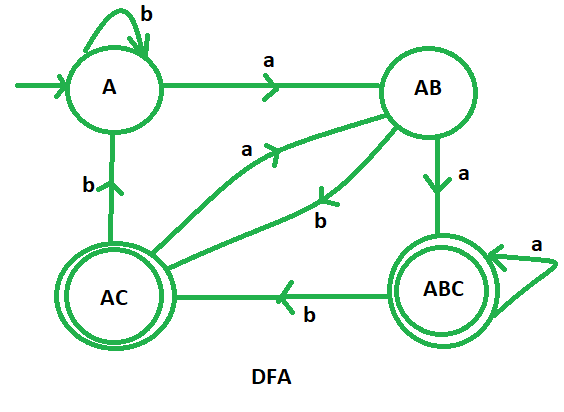# DFA of a string in which 2nd symbol from RHS is ‘a’

Draw deterministic finite automata (DFA) of the language containing the set of all strings over {a, b} in which 2nd symbol from RHS is ‘a’. The strings in which 2nd last symbol is “a” are:

aa, ab, aab, aaa, aabbaa, bbbab etc

## Input/Output

INPUT : baba

OUTPUT: NOT ACCEPTED

INPUT: aaab

OUTPUT: ACCEPTED

Constructing the DFA of the given problem directly is very complicated. So, here we are going to design the non-deterministic finite automata (NFA) and then convert it to the deterministic finite automata (DFA). The NFA of the language containing all the strings in which 2nd symbol from the RHS is “a” is:Here, A is the initial state and C is the final state. Now, we are going to construct the state transition table of the above NFA.After that we will draw the state transition table of DFA using subset configuration on the state transition table of NFA. We will mention all the possible transition for a and b.Now it’s become very easy to draw the DFA with the help of its transition table. In this DFA, we have four different states A, AB, ABC and AC, where ABC and AC are the final states and A is the initial state of the DFA.This is our required DFA of the language containing the set of all strings over {a, b} in which 2nd symbol from RHS is ‘a’.

## Transition Table

STATES INPUT (a) INPUT (b)
—> A (initial state) AB A
AB ABC* (final state) AC* (final state)
AC* (final state) AB A
ABC* (final state) ABC* (final state) AC* (final state)

## C++

 `#include ` `using` `namespace` `std;`   `// Forward declarations of state functions` `void` `stateA(string n);` `void` `stateAB(string n);` `void` `stateABC(string n);` `void` `stateAC(string n);`   `// State function for state A` `void` `stateA(string n) {` `    ``// Check if the input string is empty` `    ``if` `(n.length() == 0) {` `        ``cout << ``"string not accepted"` `<< endl;` `    ``} ``else` `{` `        ``// Check the first character of the input` `        ``if` `(n == ``'a'``) {` `            ``stateAB(n.substr(1)); ``// Call stateAB for remaining substring` `        ``} ``else` `if` `(n == ``'b'``) {` `            ``stateA(n.substr(1)); ``// Call stateA for remaining substring` `        ``}` `    ``}` `}`   `// State function for state AB` `void` `stateAB(string n) {` `    ``// Check if the input string is empty` `    ``if` `(n.length() == 0) {` `        ``cout << ``"string not accepted"` `<< endl;` `    ``} ``else` `{` `        ``// Check the first character of the input` `        ``if` `(n == ``'a'``) {` `            ``stateABC(n.substr(1)); ``// Call stateABC for remaining substring` `        ``} ``else` `if` `(n == ``'b'``) {` `            ``stateAC(n.substr(1)); ``// Call stateAC for remaining substring` `        ``}` `    ``}` `}`   `// State function for state ABC` `void` `stateABC(string n) {` `    ``// Check if the input string is empty` `    ``if` `(n.length() == 0) {` `        ``cout << ``"string accepted"` `<< endl;` `    ``} ``else` `{` `        ``// Check the first character of the input` `        ``if` `(n == ``'a'``) {` `            ``stateABC(n.substr(1)); ``// Call stateABC for remaining substring` `        ``} ``else` `if` `(n == ``'b'``) {` `            ``stateAC(n.substr(1)); ``// Call stateAC for remaining substring` `        ``}` `    ``}` `}`   `// State function for state AC` `void` `stateAC(string n) {` `    ``// Check if the input string is empty` `    ``if` `(n.length() == 0) {` `        ``cout << ``"string accepted"` `<< endl;` `    ``} ``else` `{` `        ``// Check the first character of the input` `        ``if` `(n == ``'a'``) {` `            ``stateAB(n.substr(1)); ``// Call stateAB for remaining substring` `        ``} ``else` `if` `(n == ``'b'``) {` `            ``stateA(n.substr(1)); ``// Call stateA for remaining substring` `        ``}` `    ``}` `}`   `int` `main() {` `    ``string n;` `    ``cin >> n; ``// Take input string from user` `    ``stateA(n); ``// Call stateA to check the input` `    ``return` `0;` `}`   `// This Code is Contributed by Shivam Tiwari`

## Java

 `import` `java.util.Scanner;`   `public` `class` `StateMachine {`   `    ``// Forward declarations of state functions` `    ``static` `void` `stateA(String n) {` `        ``// Check if the input string is empty` `        ``if` `(n.length() == ``0``) {` `            ``System.out.println(``"string not accepted"``);` `        ``} ``else` `{` `            ``// Check the first character of the input` `            ``if` `(n.charAt(``0``) == ``'a'``) {` `                ``stateAB(n.substring(``1``)); ``// Call stateAB for the remaining substring` `            ``} ``else` `if` `(n.charAt(``0``) == ``'b'``) {` `                ``stateA(n.substring(``1``)); ``// Call stateA for the remaining substring` `            ``}` `        ``}` `    ``}`   `    ``static` `void` `stateAB(String n) {` `        ``// Check if the input string is empty` `        ``if` `(n.length() == ``0``) {` `            ``System.out.println(``"string not accepted"``);` `        ``} ``else` `{` `            ``// Check the first character of the input` `            ``if` `(n.charAt(``0``) == ``'a'``) {` `                ``stateABC(n.substring(``1``)); ``// Call stateABC for the remaining substring` `            ``} ``else` `if` `(n.charAt(``0``) == ``'b'``) {` `                ``stateAC(n.substring(``1``)); ``// Call stateAC for the remaining substring` `            ``}` `        ``}` `    ``}`   `    ``static` `void` `stateABC(String n) {` `        ``// Check if the input string is empty` `        ``if` `(n.length() == ``0``) {` `            ``System.out.println(``"string accepted"``);` `        ``} ``else` `{` `            ``// Check the first character of the input` `            ``if` `(n.charAt(``0``) == ``'a'``) {` `                ``stateABC(n.substring(``1``)); ``// Call stateABC for the remaining substring` `            ``} ``else` `if` `(n.charAt(``0``) == ``'b'``) {` `                ``stateAC(n.substring(``1``)); ``// Call stateAC for the remaining substring` `            ``}` `        ``}` `    ``}`   `    ``static` `void` `stateAC(String n) {` `        ``// Check if the input string is empty` `        ``if` `(n.length() == ``0``) {` `            ``System.out.println(``"string accepted"``);` `        ``} ``else` `{` `            ``// Check the first character of the input` `            ``if` `(n.charAt(``0``) == ``'a'``) {` `                ``stateAB(n.substring(``1``)); ``// Call stateAB for the remaining substring` `            ``} ``else` `if` `(n.charAt(``0``) == ``'b'``) {` `                ``stateA(n.substring(``1``)); ``// Call stateA for the remaining substring` `            ``}` `        ``}` `    ``}`   `    ``public` `static` `void` `main(String[] args) {` `        ``Scanner scanner = ``new` `Scanner(System.in);` `        ``System.out.print(``"Enter the string: "``);` `        ``if` `(scanner.hasNext()) {` `            ``String n = scanner.next(); ``// Take input string from the user` `            ``stateA(n); ``// Call stateA to check the input` `        ``} ``else` `{` `            ``System.out.println(``"No input provided."``);` `        ``}` `        ``scanner.close();` `    ``}` `}`   `// This Code is Contributed by Shivam Tiwari`

## Python3

 `def` `stateA(n):` `    ``#if length found 0 ` `    ``#print not accepted` `    ``if` `(``len``(n)``=``=``0``):` `        ``print``("string ``not` `accepted")` `    ``else``:    ` `        `  `        ``#if at index 0 ` `        ``#'a' found call ` `        ``#function stateAB ` `        ``if``(n[``0``]``=``=``'a'``):` `            ``stateAB(n[``1``:])` `            `  `        ``#else if 'b' found ` `        ``#call function A.    ` `        ``elif` `(n[``0``]``=``=``'b'``):` `            ``stateA(n[``1``:])` `        `  `def` `stateAB(n):` `    ``#if length found 0 ` `    ``#print not accepted` `    ``if` `(``len``(n)``=``=``0``):` `        ``print``("string ``not` `accepted")` `    ``else``:` `        `  `        ``#if at index 0 ` `        ``#'a' found call ` `        ``#function stateABC` `        ``if``(n[``0``]``=``=``'a'``):` `            ``stateABC(n[``1``:])` `            `  `        ``#else if 'b' found ` `        ``#call function AC.     ` `        ``elif` `(n[``0``]``=``=``'b'``):` `            ``stateAC(n[``1``:]) ` `        `  `def` `stateABC(n):` `    ``#if length found 0 ` `    ``#print accepted` `    ``if` `(``len``(n)``=``=``0``):` `        ``print``("string accepted")` `    ``else``:` `        `  `        ``#if at index 0 ` `        ``#'a' found call ` `        ``#function stateABC` `        ``if``(n[``0``]``=``=``'a'``):` `            ``stateABC(n[``1``:])` `            `  `        ``#else if 'b' found ` `        ``#call function AC.     ` `        ``elif` `(n[``0``]``=``=``'b'``):` `            ``stateAC(n[``1``:])` `        `  `def` `stateAC(n):` `    ``#if length found 0 ` `    ``#print accepted` `    ``if` `(``len``(n)``=``=``0``):` `        ``print``("string accepted")` `    ``else``:` `        ``#if at index 0 ` `        ``#'a' found call ` `        ``#function stateAB` `        ``if``(n[``0``]``=``=``'a'``):` `            ``stateAB(n[``1``:])` `            `  `        ``#else if 'b' found ` `        ``#call function A.     ` `        ``elif` `(n[``0``]``=``=``'b'``):` `            ``stateA(n[``1``:])        ` `  `    `#take string input` `n``=``input``()`   `#call stateA` `#to check the input` `stateA(n)`

## Output

Input: aaab

Output: string accepted

Input: baba

Output: string not accepted

### Q.1: Why is it required to develop a non-deterministic finite automaton (NFA) before building a DFA for this problem?

For languages with complex patterns, designing an NFA first simplifies DFA construction. NFAs make language properties easier to visualize and work with by providing more flexibility. The translation from NFA to DFA ensures determinism and organizes problem-solving.

### Q.2: Can this method work for languages with more sophisticated patterns and conditions?

Yes, languages with more complex patterns and circumstances can use this NFA-to-DFA method. It breaks down the challenge into small steps and gives a systematic DFA for such languages. As language complexity develops, state transition tables and the DFA may grow and require careful design.

## Conclusion

Non-deterministic finite automata (NFA) simplifies building deterministic finite automata (DFA) for languages with specified patterns, such as strings with the 2nd symbol from the right being ‘a’. This method simplifies and organizes DFA construction. Automata theory benefits from this method for representing and processing complex linguistic patterns.